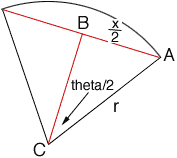Quandaries and Queries To Whom it concerns: Can you help me find a formula: find the length of a circle's chord with a known arc length and radius/diameter lengths. The best I have found so far is: there is NO formula for this. Is this true? We've somehow got to at least accurately approximate it. (example: an arc length is This will be used in a homebuilding project and I could use an answer ASAP if someone there knows this. Thanks in advance so much. BES a homebuilder Hi, A relationship involving the length of an arc (a), the radius of the circle (r) and the angle at the center of the circle (theta) is a = rtheta where theta is measured in radians. Let x be the length of the chord and bisect the angle at the center of the circle to get a right triangle ABC as in the diagram.Since triangle ABC is a right triangle  x/2 = rsin( theta /2) but a = rtheta so x = 2rsin( a/2r ) Penny